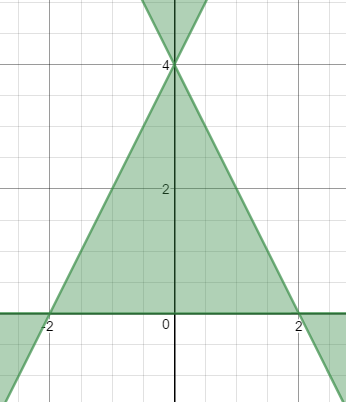Games
Problems
Go Pro!A follow-up question from this year's Christmas Graph Post: "You said we should be able to factor equation T (the Christmas tree outline equation). How?" - Kevin from Nebraska

Hi Kevin, first of all, for anyone who doesn't want to go find the equation in the other post, here it is:

Let T be the equation: 4x2y - y3 + 8y2 - 16y ≤ 0

The first step in factoring this should be easy; every term on the left has a y in it, so we can factor that out:

y(4x2 - y2 + 8y - 16)

The next step is to split 4x2 - y2 + 8y - 16 into groups, in the hope that we can find a grouping that will help us factor it further. Grouping is not always a cut-and-dried process; sometimes it's easy to find a grouping that helps, sometimes it's hard. And, of course, sometimes it's impossible, which makes the process both frustrating (when you can't find a grouping that works) and satisfying (when you do!).

Typically, if I have four terms, the first thing I try is to split it into pairs, and factor each of the pairs:

(4x2 - y2) + (8y - 16)
(2x - y)(2x + y) + 8(y - 2)

If the (y - 2) on the right matched either of the binomials on the left, we'd be able to factor this some more. Alas, no such luck. Let's try another grouping:

(4x2 -16) - (y2 - 8y)
4(x - 2)(x + 2) - y(y - 8)

Still no joy. Let's try another grouping:

(4x2 + 8y) - (y2 +16)

This one is also useless, because we can't factor the second group at all. At this point, I start re-evaluating my method; maybe grouping in pairs isn't the right way to go. Maybe I should create groups that are mismatched in size. For example:

4x2 - (y2 - 8y + 16)

Suddenly, I realize, "Hey! That thing in the parentheses is a perfect square!"

4x2 - (y - 4)2

Wonderful! This is a difference of squares!

[2x + (y - 4)][2x - (y - 4)]
(2x + y - 4)(2x - y + 4)

So the full factorization is:

y(2x + y - 4)(2x - y + 4) ≤ 0

Now you can go back to the page for the Christmas tree graph and see why this graphs as shown at the top of this post.Like us on Facebook to get updates about new resources